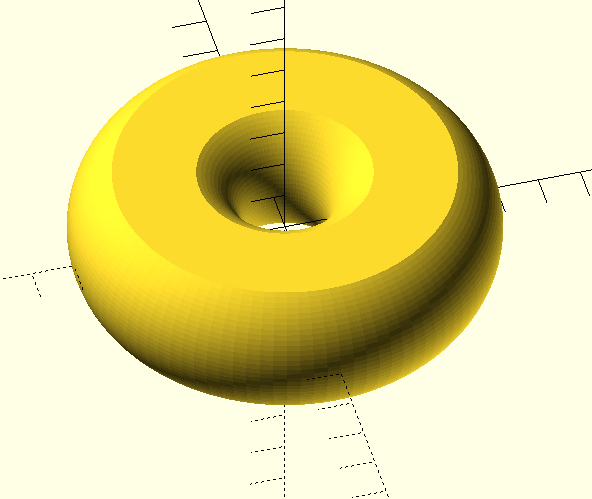### Re: Error while rendering; what's wrong?G
Fri, Jan 14, 2022 1:53 PM

This works now:

module ring(d,b,r)
{
intersection()
{
union(){
rotate_extrude(convexity = 10,\$fn = 100)
translate([max(d/2,r+.001), 0])
circle(r = r,\$fn=100);
}
cylinder(d=d+r*2, h=b, center=true,\$fn = 100);
}
}
ring(56,40,30);

This works now: module ring(d,b,r) { intersection() { union(){ rotate_extrude(convexity = 10,\$fn = 100) translate([max(d/2,r+.001), 0]) circle(r = r,\$fn=100); } cylinder(d=d+r*2, h=b, center=true,\$fn = 100); } } ring(56,40,30);R
richard@itrgmbh.de
Fri, Jan 14, 2022 2:13 PM

yes. it’s working now! I have to think about, why!?

I should name the module“donut” :D

like this with parameters:

ring(60,35,20);G
Fri, Jan 14, 2022 2:15 PM

Personally I would use external dimensions to define the object:

module ring(do,di,rh){
intersection(){
rotate_extrude(convexity = 10,\$fn = 100)
translate([(do+di)/4+.001, 0])
circle(d = (do-di)/2,\$fn=100);
cylinder(d=do, h=rh, center=true,\$fn = 100);
}
}
ring(60,20,18);

Personally I would use external dimensions to define the object: module ring(do,di,rh){ intersection(){ rotate_extrude(convexity = 10,\$fn = 100) translate([(do+di)/4+.001, 0]) circle(d = (do-di)/2,\$fn=100); cylinder(d=do, h=rh, center=true,\$fn = 100); } } ring(60,20,18);